### Practice Set 3.4 Circle Class 10th Mathematics Part 2 MHB Solution

Practice Set 3.4

1. In figure 3.56, in a circle with centre O, length of chord AB is equal to the radius of…
2. In figure 3.57, is cyclic. side PQ ≅ side RQ. ∠ PSR = 110°, Find-(1) measure of ∠…
3. is cyclic, ∠R = (5x - 13)°, ∠N = (4x + 4)°. Find measures of angle r and triangle…
4. In figure 3.58, seg RS is a diameter of the circle with centre O. Point T lies in the…
5. Prove that, any rectangle is a cyclic quadrilateral.
6. In figure 3.59, altitudes YZ and XT of∆ WXY intersect at P. Prove that, x^{w}sum t (1)…
7. In figure 3.60, m(arc NS) = 125°, m(arc EF) = 37°, find the measure ∠ NMS.…
8. In figure 3.61, chords AC and DE intersect at B. If ∠ ABE = 108°, m(arc AE) = 95°, find…

###### Practice Set 3.4
Question 1.

In figure 3.56, in a circle with centre O, length of chord AB is equal to the radius of the circle. Find measure of each of the following.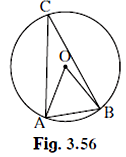(1) ∠ AOB (2) ∠ ACB

(3) arc AB (4) arc ACB.

(1)In ∆AOB,

AB = OA = OB = radius of circle

⇒ ∆AOB is an equilateral triangle

∠ AOB + ∠ ABO + ∠ BAO = 180° {Angle sum property}

⇒ 3∠ AOB = 180° {All the angles are equal}

∠ AOB = 60°

(2)∠ AOB = 2 × ∠ ACB {The measure of an inscribed angle is half the measure of the arc intercepted by it.}

⇒∠ ACB = 30°

(3)∠ AOB = 60°

⇒arc(AB) = 60° {The measure of a minor arc is the measure of its central angle.}

(4) Using Measure of a major arc = 360°- measure of its corresponding minor arc

⇒arc(ACB) = 360° - arc(AB)

⇒arc(ACB) = 360° - 60° = 300°

Question 2.

In figure 3.57,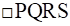is cyclic. side PQ ≅ side RQ. ∠ PSR = 110°, Find-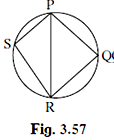(1) measure of ∠ PQR

(2) m(arc PQR)

(3) m(arc QR)

(4) measure of ∠ PRQ

(1) Given PQRS is a cyclic quadrilateral.

∵Opposite angles of a cyclic quadrilateral are supplementary

⇒∠ PSR + ∠ PQR = 180°

⇒∠ PQR = 180° - 110°

⇒∠ PQR = 70°

(2)2 × ∠ PQR = m(arc PR){The measure of an inscribed angle is half the measure of the arc intercepted by it.}

m(arc PR) = 140°

⇒m(arc PQR) = 360° -140° = 220° {Using Measure of a major arc = 360°- measure of its corresponding minor arc}

(3)side PQ ≅ side RQ

∴m(arc PQ) = m(arc RQ){Corresponding arcs of congruent chords of a circle (or congruent circles) are congruent}

⇒m(arc PQR) = m(arc PQ) + m(arc RQ)

⇒m(arc PQR) = 2 × m(arc PQ)

⇒m(arc PQ) = 110°

(4)In ∆ PQR,

∠ PQR + ∠ QRP + ∠ RPQ = 180°{Angle sum property}

⇒∠ PRQ + ∠ RPQ = 180° - ∠ PQR

⇒ 2∠ PRQ = 180° - 70° {∵side PQ ≅ side RQ}

⇒∠ PRQ = 55°

Question 3.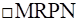is cyclic, ∠R = (5x - 13)°, ∠N = (4x + 4)°. Find measures of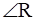and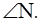Given MRPN is a cyclic quadrilateral.

⇒∠ R + ∠ N = 180° {Using Opposite angles of a cyclic quadrilateral are supplementary}

⇒ (5x - 13)° + (4x + 4)° = 180°

⇒9x - 9 = 180°

⇒ x – 1 = 20°

⇒ x = 21°

∠R = (5x - 13)° = 5 × 21 – 13 = 105 – 13 = 92°

∠N = (4x + 4)° = 4 × 21 + 4 = 84 + 4 = 88°

Question 4.

In figure 3.58, seg RS is a diameter of the circle with centre O. Point T lies in the exterior of the circle. Prove that ∠ RTS is an acute angle.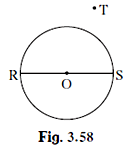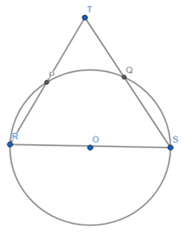Given RS is the diameter

⇒ ∠ ROS = 180°

m(arc RS) = 180°

Now, ∠ RTS is an external angle.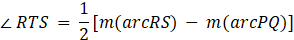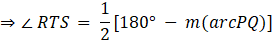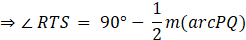Hence, ∠ RTS is an acute angle.

Question 5.

Prove that, any rectangle is a cyclic quadrilateral.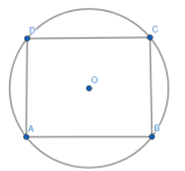In ABCD,

∠A = 90°{∵ angle of a rectangle is 90°.}

∠C = 90° {opposite angles are equals}

⇒∠ A + ∠ C = 180°

If opposite angles are supplementary, the quadrilateral is cyclic.

∴ ABCD is cyclic.

Question 6.

In figure 3.59, altitudes YZ and XT of

∆ WXY intersect at P. Prove that,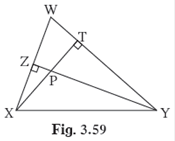(1)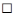WZPT is cyclic.

(2) Points X, Z, T, Y are concyclic.

(1)In WZPT,

∠ WZP = ∠ WTP = 90° {YZ and XT are the altitudes}

If a pair of opposite angles of a quadrilateral is supplementary, then the

⇒ WZPT is cyclic.

(2)∵ X, Z,T,Y lie on same circle, ∴ they are concyclic.

Question 7.

In figure 3.60, m(arc NS) = 125°, m(arc EF) = 37°, find the measure ∠ NMS.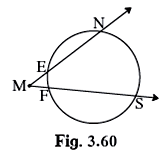Given m(arc NS) = 125°, m(arc EF) = 37°

Also, ∠ NMS is an external angle, so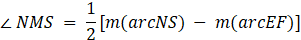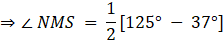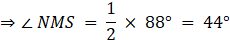Question 8.

In figure 3.61, chords AC and DE intersect at B. If ∠ ABE = 108°, m(arc AE) = 95°, find m(arc DC).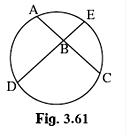Given ∠ ABE = 108°, m(arc AE) = 95°

Using the property of the secant,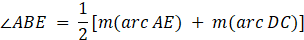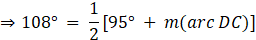⇒m(arc DC) = 108° × 2 – 95°

⇒m(arc DC) = 121°

## PDF FILE TO YOUR EMAIL IMMEDIATELY PURCHASE NOTES & PAPER SOLUTION. @ Rs. 50/- each (GST extra)

SUBJECTS

HINDI ENTIRE PAPER SOLUTION

MARATHI PAPER SOLUTION
SSC MATHS I PAPER SOLUTION
SSC MATHS II PAPER SOLUTION
SSC SCIENCE I PAPER SOLUTION
SSC SCIENCE II PAPER SOLUTION
SSC ENGLISH PAPER SOLUTION
SSC & HSC ENGLISH WRITING SKILL
HSC ACCOUNTS NOTES
HSC OCM NOTES
HSC ECONOMICS NOTES
HSC SECRETARIAL PRACTICE NOTES

2019 Board Paper Solution

HSC ENGLISH SET A 2019 21st February, 2019

HSC ENGLISH SET B 2019 21st February, 2019

HSC ENGLISH SET C 2019 21st February, 2019

HSC ENGLISH SET D 2019 21st February, 2019

SECRETARIAL PRACTICE (S.P) 2019 25th February, 2019

HSC XII PHYSICS 2019 25th February, 2019

CHEMISTRY XII HSC SOLUTION 27th, February, 2019

OCM PAPER SOLUTION 2019 27th, February, 2019

HSC MATHS PAPER SOLUTION COMMERCE, 2nd March, 2019

HSC MATHS PAPER SOLUTION SCIENCE 2nd, March, 2019

SSC ENGLISH STD 10 5TH MARCH, 2019.

HSC XII ACCOUNTS 2019 6th March, 2019

HSC XII BIOLOGY 2019 6TH March, 2019

HSC XII ECONOMICS 9Th March 2019

SSC Maths I March 2019 Solution 10th Standard11th, March, 2019

SSC MATHS II MARCH 2019 SOLUTION 10TH STD.13th March, 2019

SSC SCIENCE I MARCH 2019 SOLUTION 10TH STD. 15th March, 2019.

SSC SCIENCE II MARCH 2019 SOLUTION 10TH STD. 18th March, 2019.

SSC SOCIAL SCIENCE I MARCH 2019 SOLUTION20th March, 2019

SSC SOCIAL SCIENCE II MARCH 2019 SOLUTION, 22nd March, 2019

XII CBSE - BOARD - MARCH - 2019 ENGLISH - QP + SOLUTIONS, 2nd March, 2019

HSC Maharashtra Board Papers 2020

(Std 12th English Medium)

HSC ECONOMICS MARCH 2020

HSC OCM MARCH 2020

HSC ACCOUNTS MARCH 2020

HSC S.P. MARCH 2020

HSC ENGLISH MARCH 2020

HSC HINDI MARCH 2020

HSC MARATHI MARCH 2020

HSC MATHS MARCH 2020

SSC Maharashtra Board Papers 2020

(Std 10th English Medium)

English MARCH 2020

HindI MARCH 2020

Hindi (Composite) MARCH 2020

Marathi MARCH 2020

Mathematics (Paper 1) MARCH 2020

Mathematics (Paper 2) MARCH 2020

Sanskrit MARCH 2020

Important-formula

THANKS